#### Differential rotations

To express the change in the moment of momentum in detail, the concept of a differential rotation is needed. In the plane, it is straightforward to define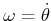; however, for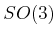, it is more complicated. One choice is to define derivatives with respect to yaw-pitch-roll variables, but this leads to distortions and singularities, which are problematic for the Newton-Euler formulation. Instead, a differential rotation is defined as shown in Figure 13.11. Let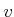denote a unit vector in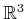, and let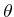denote a rotation that is analogous to the 2D case. Let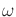denote the angular velocity vector,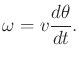(13.83)

This provides a natural expression for angular velocity.13.9 The change in a rotation matrix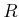with respect to time is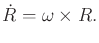(13.84)

This relationship can be used to derive expressions that relateto yaw-pitch-roll angles or quaternions. For example, using the yaw-pitch-roll matrix (3.42) the conversion fromto the change yaw, pitch, and roll angles is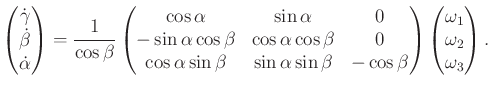(13.85)

Steven M LaValle 2020-08-14